• A binomial queue is not a heap-ordered tree, but rather a collection of heap-ordered trees, known as a forest. Each heap-ordered tree is a binomial tree. Observation : Bk consists of a root with ...
A binomial queue is not a heap-ordered tree, but rather a collection of heap-ordered trees, known as a forest.  Each heap-ordered tree is a binomial tree.
Observation :   Bk consists of a root with   k  children, which are B0,B1...Bk-1 .  Bk has exactly   2^k   nodes.  The number of nodes at depth d is  Ck d(组合数) .
Assume that there are N elements in a binomial queue, we can represent N in binary form.
typedef struct BinNode *Position;
typedef struct Collection *BinQueue;
typedef struct BinNode *BinTree;  /* missing from p.176 */

struct BinNode
{
ElementType	    Element;
Position	    LeftChild;
Position 	    NextSibling;
} ;

struct Collection
{
int	    	CurrentSize;  /* total number of nodes */
BinTree	TheTrees[ MaxTrees ];
} ;

operations:
FindMin : the number of trees is log(N+1)(下整).Traversal the root.
Merge : H1,H2
combine trees in same height and carry it.
BinQueue  Merge( BinQueue H1, BinQueue H2 )
{	BinTree T1, T2, Carry = NULL;
int i, j;
if ( H1->CurrentSize + H2-> CurrentSize > Capacity )  ErrorMessage();
H1->CurrentSize += H2-> CurrentSize;
for ( i=0, j=1; j<= H1->CurrentSize; i++, j*=2 ) {
T1 = H1->TheTrees[i]; T2 = H2->TheTrees[i]; /*current trees */
switch( 4*!!Carry + 2*!!T2 + !!T1 ) {
case 0: /* 000 */
case 1: /* 001 */  break;
case 2: /* 010 */  H1->TheTrees[i] = T2; H2->TheTrees[i] = NULL; break;
case 4: /* 100 */  H1->TheTrees[i] = Carry; Carry = NULL; break;
case 3: /* 011 */  Carry = CombineTrees( T1, T2 );
H1->TheTrees[i] = H2->TheTrees[i] = NULL; break;
case 5: /* 101 */  Carry = CombineTrees( T1, Carry );
H1->TheTrees[i] = NULL; break;
case 6: /* 110 */  Carry = CombineTrees( T2, Carry );
H2->TheTrees[i] = NULL; break;
case 7: /* 111 */  H1->TheTrees[i] = Carry;
Carry = CombineTrees( T1, T2 );
H2->TheTrees[i] = NULL; break;
} /* end switch */
} /* end for-loop */
return H1;
}

DeleteMin(O(logN)) : find the heap with the minimal element(O(logN)).remove it from the original heap H(O(1)), and its subtrees form a now binomial queue H2(O(logN)).
Finally,merge H and H2(O(logN)).
ElementType  DeleteMin( BinQueue H )
{	BinQueue DeletedQueue;
Position DeletedTree, OldRoot;
ElementType MinItem = Infinity;  /* the minimum item to be returned */
int i, j, MinTree; /* MinTree is the index of the tree with the minimum item */

if ( IsEmpty( H ) )  {  PrintErrorMessage();  return –Infinity; }

for ( i = 0; i < MaxTrees; i++) {  /* Step 1: find the minimum item */
if( H->TheTrees[i] && H->TheTrees[i]->Element < MinItem ) {
MinItem = H->TheTrees[i]->Element;  MinTree = i;    } /* end if */
} /* end for-i-loop */
DeletedTree = H->TheTrees[ MinTree ];
H->TheTrees[ MinTree ] = NULL;   /* Step 2: remove the MinTree from H => H’ */
OldRoot = DeletedTree;   /* Step 3.1: remove the root */
DeletedTree = DeletedTree->LeftChild;   free(OldRoot);
DeletedQueue = Initialize();   /* Step 3.2: create H” */
DeletedQueue->CurrentSize = ( 1<<MinTree ) – 1;  /* 2MinTree – 1 */
for ( j = MinTree – 1; j >= 0; j – – ) {
DeletedQueue->TheTrees[j] = DeletedTree;
DeletedTree = DeletedTree->NextSibling;
DeletedQueue->TheTrees[j]->NextSibling = NULL;
} /* end for-j-loop */
H->CurrentSize  – = DeletedQueue->CurrentSize + 1;
H = Merge( H, DeletedQueue ); /* Step 4: merge H’ and H” */
return MinItem;
}

【Claim】A binomial queue of N elements can be built by N successive insertions in O(N) time.
1.N+N(1/2+1/4+...+1/N)=O(N)
2.Ci=the cost of i-th insertion
Φi=the number of heap
Φi - Φi-1 =2-c(insert a node need 1 cost, and carry need c-1. Every time carrying, we lose a heap)
Ci+Φi - Φi-1 = 2
T armotize = O(1)
展开全文• The binomial coefficient C(n, k) has been extensively studied for its importance in combinatorics. Binomial coefficients can be recursively defined as follows: C(n, 0) = C(n, n) = 1 for all n > 0; C...as c each
• // BinomialQueue class // // CONSTRUCTION: with no parameters or a single item // // ******************PUBLIC OPERATIONS********************* // void insert( x ) --> Insert x // Comparable ...
package heap;// BinomialQueue class
//
// CONSTRUCTION: with no parameters or a single item
//
// ******************PUBLIC OPERATIONS*********************
// void insert( x )       --> Insert x
// Comparable deleteMin( )--> Return and remove smallest item
// Comparable findMin( )  --> Return smallest item
// boolean isEmpty( )     --> Return true if empty; else false
// void makeEmpty( )      --> Remove all items
// vod merge( rhs )       --> Absord rhs into this heap
// ******************ERRORS********************************
// Throws UnderflowException as appropriate

/**
* Implements a binomial queue.
* Note that all "matching" is based on the compareTo method.
*
* @author Mark Allen Weiss
*/
public final class BinomialQueue<AnyType extends Comparable<? super AnyType>> {
/**
* Construct the binomial queue.
*/
public BinomialQueue() {
theTrees = new BinNode[DEFAULT_TREES];
makeEmpty();
}

/**
* Construct with a single item.
*/
public BinomialQueue(AnyType item) {
currentSize = 1;
theTrees = new BinNode;
theTrees = new BinNode<>(item, null, null);
}

private void expandTheTrees(int newNumTrees) {
BinNode<AnyType>[] old = theTrees;
int oldNumTrees = theTrees.length;

theTrees = new BinNode[newNumTrees];
for (int i = 0; i < Math.min(oldNumTrees, newNumTrees); i++)
theTrees[i] = old[i];
for (int i = oldNumTrees; i < newNumTrees; i++)
theTrees[i] = null;
}

/**
* Merge rhs into the priority queue.
* rhs becomes empty. rhs must be different from this.
*
* @param rhs the other binomial queue.
*/
public void merge(BinomialQueue<AnyType> rhs) {
if (this == rhs)    // Avoid aliasing problems
return;

currentSize += rhs.currentSize;

if (currentSize > capacity()) {
int newNumTrees = Math.max(theTrees.length, rhs.theTrees.length) + 1;
expandTheTrees(newNumTrees);
}

BinNode<AnyType> carry = null;
for (int i = 0, j = 1; j <= currentSize; i++, j *= 2) {
BinNode<AnyType> t1 = theTrees[i];
BinNode<AnyType> t2 = i < rhs.theTrees.length ? rhs.theTrees[i] : null;

int whichCase = t1 == null ? 0 : 1;
whichCase += t2 == null ? 0 : 2;
whichCase += carry == null ? 0 : 4;

switch (whichCase) {
case 0: /* No trees */
case 1: /* Only this */
break;
case 2: /* Only rhs */
theTrees[i] = t2;
rhs.theTrees[i] = null;
break;
case 4: /* Only carry */
theTrees[i] = carry;
carry = null;
break;
case 3: /* this and rhs */
carry = combineTrees(t1, t2);
theTrees[i] = rhs.theTrees[i] = null;
break;
case 5: /* this and carry */
carry = combineTrees(t1, carry);
theTrees[i] = null;
break;
case 6: /* rhs and carry */
carry = combineTrees(t2, carry);
rhs.theTrees[i] = null;
break;
case 7: /* All three */
theTrees[i] = carry;
carry = combineTrees(t1, t2);
rhs.theTrees[i] = null;
break;
}
}

for (int k = 0; k < rhs.theTrees.length; k++)
rhs.theTrees[k] = null;
rhs.currentSize = 0;
}

/**
* Return the result of merging equal-sized t1 and t2.
*/
private BinNode<AnyType> combineTrees(BinNode<AnyType> t1, BinNode<AnyType> t2) {
if (t1.element.compareTo(t2.element) > 0)
return combineTrees(t2, t1);
t2.nextSibling = t1.leftChild;
t1.leftChild = t2;
return t1;
}

/**
* Insert into the priority queue, maintaining heap order.
* This implementation is not optimized for O(1) performance.
*
* @param x the item to insert.
*/
public void insert(AnyType x) {
merge(new BinomialQueue<>(x));
}

/**
* Find the smallest item in the priority queue.
*
* @return the smallest item, or throw UnderflowException if empty.
*/
public AnyType findMin() {
if (isEmpty())
throw new UnderflowException();

return theTrees[findMinIndex()].element;
}

/**
* Find index of tree containing the smallest item in the priority queue.
* The priority queue must not be empty.
*
* @return the index of tree containing the smallest item.
*/
private int findMinIndex() {
int i;
int minIndex;

for (i = 0; theTrees[i] == null; i++)
;

for (minIndex = i; i < theTrees.length; i++)
if (theTrees[i] != null &&
theTrees[i].element.compareTo(theTrees[minIndex].element) < 0)
minIndex = i;

return minIndex;
}

/**
* Remove the smallest item from the priority queue.
*
* @return the smallest item, or throw UnderflowException if empty.
*/
public AnyType deleteMin() {
if (isEmpty())
throw new UnderflowException();

int minIndex = findMinIndex();
AnyType minItem = theTrees[minIndex].element;

BinNode<AnyType> deletedTree = theTrees[minIndex].leftChild;

// Construct H''
BinomialQueue<AnyType> deletedQueue = new BinomialQueue<>();
deletedQueue.expandTheTrees(minIndex);

deletedQueue.currentSize = (1 << minIndex) - 1;
for (int j = minIndex - 1; j >= 0; j--) {
deletedQueue.theTrees[j] = deletedTree;
deletedTree = deletedTree.nextSibling;
deletedQueue.theTrees[j].nextSibling = null;
}

// Construct H'
theTrees[minIndex] = null;
currentSize -= deletedQueue.currentSize + 1;

merge(deletedQueue);

return minItem;
}

/**
* Test if the priority queue is logically empty.
*
* @return true if empty, false otherwise.
*/
public boolean isEmpty() {
return currentSize == 0;
}

/**
* Make the priority queue logically empty.
*/
public void makeEmpty() {
currentSize = 0;
for (int i = 0; i < theTrees.length; i++)
theTrees[i] = null;
}

private static class BinNode<AnyType> {
// Constructors
BinNode(AnyType theElement) {
this(theElement, null, null);
}

BinNode(AnyType theElement, BinNode<AnyType> lt, BinNode<AnyType> nt) {
element = theElement;
leftChild = lt;
nextSibling = nt;
}

AnyType element;     // The data in the node
BinNode<AnyType> leftChild;   // Left child
BinNode<AnyType> nextSibling; // Right child
}

private static final int DEFAULT_TREES = 1;

private int currentSize;                // # items in priority queue
private BinNode<AnyType>[] theTrees;  // An array of tree roots

/**
* Return the capacity.
*/
private int capacity() {
return (1 << theTrees.length) - 1;
}

public static void main(String[] args) {
int numItems = 10000;
BinomialQueue<Integer> h = new BinomialQueue<>();
BinomialQueue<Integer> h1 = new BinomialQueue<>();
int i = 37;

System.out.println("Starting check.");

for (i = 37; i != 0; i = (i + 37) % numItems)
if (i % 2 == 0)
h1.insert(i);
else
h.insert(i);

h.merge(h1);
for (i = 1; i < numItems; i++)
if (h.deleteMin() != i)
System.out.println("Oops! " + i);

System.out.println("Check done.");
}
}

展开全文• In mathematics, the binomial theorem is an important formula giving the expansion of powers of sums. Its simplest version states that for any real or complex numbers x and y, and any nonnegative ...
• Binomial Coeffcients Time Limit: 1000MS Memory limit: 65536K 题目描述 输入 输出 示例输入 3 1 1 10 2 954 723 示例输出 1 45 3557658当时刚看到这道题的时候，以为是道高精度的题...


Binomial Coeffcients

Time Limit: 1000MS Memory limit: 65536K

题目描述输入输出示例输入

3
1 1
10 2
954 723

示例输出

1
45
3557658当时刚看到这道题的时候，以为是道高精度的题，做一半天没弄出来。之后才知道是杨辉三角（组合数）。

#include<stdio.h>
#include<string.h>
#include<math.h>
int a;
int main()
{
int t,n,m,i,j;
memset(a,0,sizeof(a));
a=1;
for(i=1; i<=1000; i++)
{
a[i]=1;
for(j=1; j<=i; j++)
{
a[i][j]=a[i-1][j]+a[i-1][j-1];
if(a[i][j]>10000003)
a[i][j]=a[i][j]-10000003;
}
}
scanf("%d",&t);
while(t--)
{
scanf("%d%d",&n,&m);
printf("%d\n",a[n][m]);
}
return 0;
}


转载于:https://www.cnblogs.com/guoyongzhi/p/3233002.html
展开全文• Lazy Binomial HeapsmotivationMeldable Priority Queue更多细节——Recap from last timelazy binomial heapwhere we standcoalesce stepThe story so far后记 motivation 为什么我们需要Binomial heaps(二项堆)？ ...


Lazy Binomial Heaps
motivationMeldable Priority Queue更多细节——Recap from last timelazy binomial heapwhere we standcoalesce stepThe story so far
后记

motivation
为什么我们需要Binomial heaps(二项堆)？ Binary heaps(二叉堆) 实现的优先队列就已经有了 O(logn) 复杂度的 enqueue(insert) 和 extractMin(deleteMin)，findMin O(1), 实际运作过程中就已经很快了，为何还需要其他 堆 呢？
因为很多图算法都不止依赖于优先队列的这两种操作，还依赖于它提供的额外操作: meld (merge or union)——合并两个优先队列 (MSTs via Cheriton-Tarjan) ，decreaseKey——提高队列内某个元素的priority (Shortest paths via Dijkstra)，add_to_all——add $\Delta$k to the keys of each element in pq.
Meldable Priority Queue
试想如何用二叉堆实现的优先队列实现两个 pq 的合并呢？可以两个混在一块 heapify，O(n) 看起来还可以；或是调用接口 A.insert(B.deleteMin())，O(nlogn) 看起来就不太行。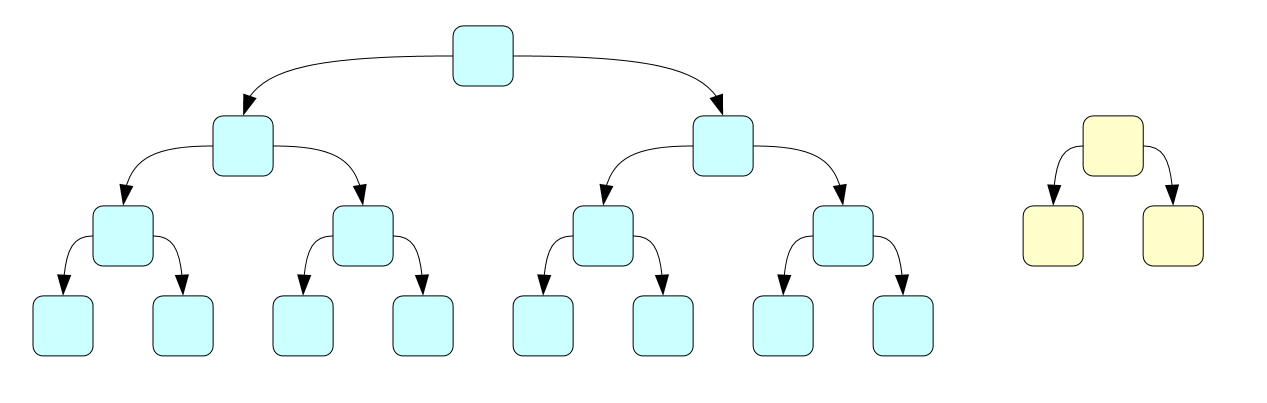而如果我们用二进制来表示这两个 pq，那么合并相当于 二进制加法，这样复杂度就变成了 O(logn+logm)。在这种思路下，我们把 n 和 m 表示成两个 packets collection每一个 packet 2 的幂次大小，用来存数。然后用指针把这些 packet 和 node link，这样合并就变成了：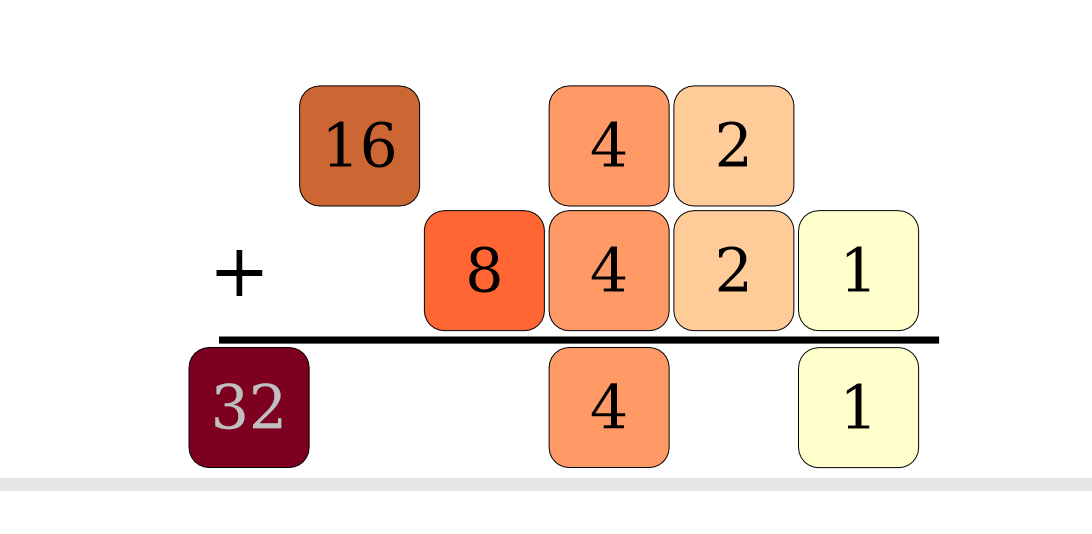更多细节——Recap from last time
我们在算法设计搬运（3）——Heaps这部分内容里讲了 binomial tree 和 binomial heaps 的操作及性能分析。因为我们在这里已经不是在搬运 570 的内容了，而是在补充 Victor 在 570 上略过的知识，为它们做详细补充，更详细的的披露细节，首先来是 binomial tree 的性质。
Properties of Bk
含有 2k 个 nodesheight 为 k第

i

i

层有

(

i

k

)

(_i^k)

个 nodesrank(root(Bk)) = rank(Bk) = k根的孩子从左到右为 Bk-1, Bk-2,…, B0.
而 binomial heap H 则是 binomial tree 的 set，且 set 中的 B 满足 min-heap 的性质。对于 eager binomial heap，我们还要求 B 的 rank 唯一。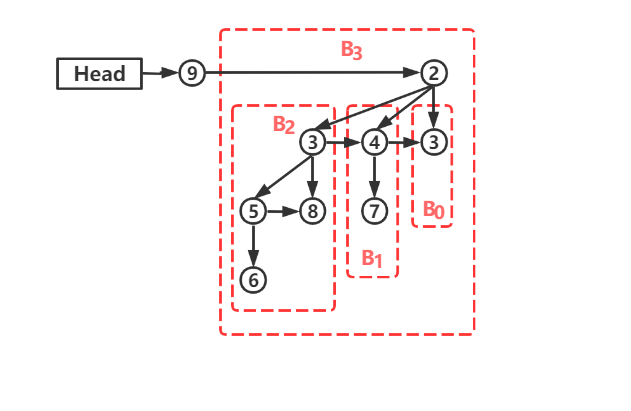然后之前没有涉及到 meld 操作，那么对于 eager binomial heap，meld(H1,H2): H2 order 低到高往 H1 里添加，扫描对应位置，rank 不唯一则比较根大小，link，order+1，直至 rank 唯一，直到全部添加完，O(log n)。另外，insert(H,v) 的实现实际上就是 MAKE-HEAP(v), 在对这个只含单一节点的 heap 和 H 进行 meld。
其他操作和复杂度分析详见算法设计搬运（3）——Heaps 和 Potential Method —— amortized analysis。
lazy binomial heap
where we stand
在继续下面的内容之前我们先看一下 eager binomial heap 的性能表现。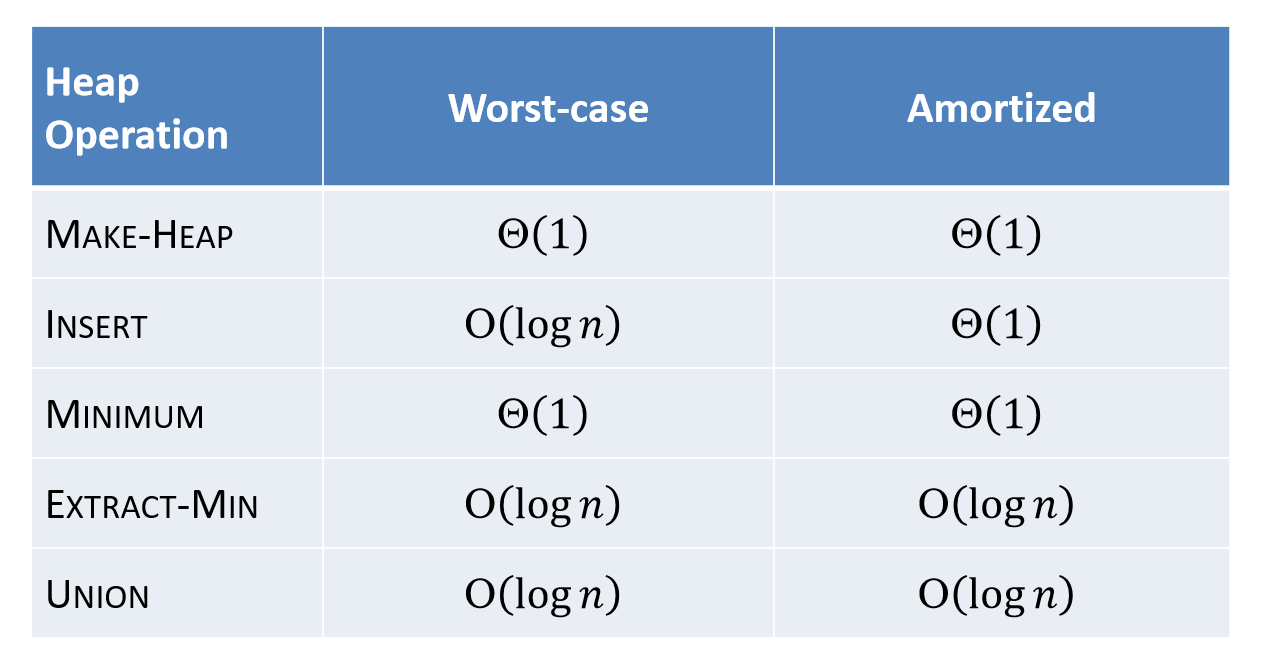(MINIMUM 因为存了指针，在 insert 和 extractMin 时顺便 update，所以

Θ

(

1

)

\Theta(1)

.）
根据“对于 comparison-based sort 需要

Ω

(

n

log

⁡

n

)

\Omega(n\log n)

”这个定理，看看我们现在的处境可以发现，能优化的地方不多。我们不可能让 insert 和 extractMin 都优化到更快——指都小于O(logn)，但是却可以考虑使其中一项操作变得更快。
那么就考虑优化 insert 吧！看看能不能使在 worst-case 也可以

O

(

1

)

O(1)

，毕竟删前总要插。那么因为 insert 或 enqueue 是用 meld 实现的，那么 meld 也必须

O

(

1

)

O(1)

了。
问题：为什么我们在插入时就要维护呢，我们都不一定要对新插入进来的节点做任何操作？
思路：我们索性 insert 或 meld 的时候就只维护 min 指针，然后直接把 H2 直接连进来，复杂度

O

(

1

)

O(1)

，然后如果有其他要维护的事情就全交给 extractMin 吧！
例： 对于 lazy binomial heap 插入 4, 7, 3, 6, 5. (In practice，实现是双向循环链表)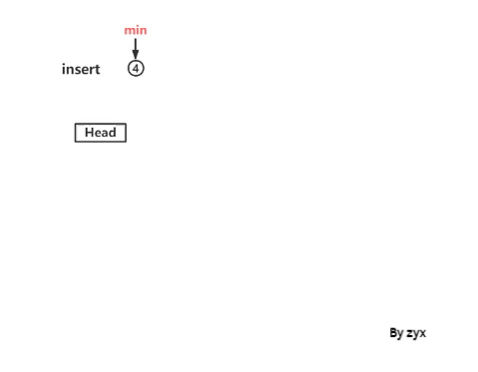试想一下这样实现 lazy meld 之后，extractMin 会怎样？
先 remove min 指针所指向 binomial tree 的 root，把 Bk 分裂成 BK-1…B0 再 lazy meld 回来再 update min 指针
可达鸭眉头一皱，发现在最后 update 时的复杂度取决于 此时 heap 里面 tree 的个数，O(t)。而t = O(n)，于是 extractMin O(n)。
像极了中学假期在家的你。白天父母外出工作，于是玩的时候不需要考虑收拾可以随意地造。其余的事情就等着之后掐着父母下班的点再把一切收拾干净，装作这一天平静度过什么都没有发生一样。恭喜你年纪轻轻就已经是个优化带师，成功地把 van 的复杂度降到了最低。
接着来解决 extractMin 变 O(n) 的问题。分析可知这是由于我们不再限制 Bk 的order唯一，meld 时只单纯 link。那么就考虑我们对应 lazy meld，在 extractMin 时把该合并的合并了，保证 order 唯一这样我们的树的个数就变 O(log n)。
这个在 extractMin 时重新合并 tree 使 order 再次唯一的操作在某些教材上称之为 coalesce step.
coalesce step
简言之， coalesce step 就是在extractMin之后，update min 指针之前合并所有order相同的 tree 使 order 唯一。
考虑到同 order 合并，且 order 破碎，使用基排序来高效合并。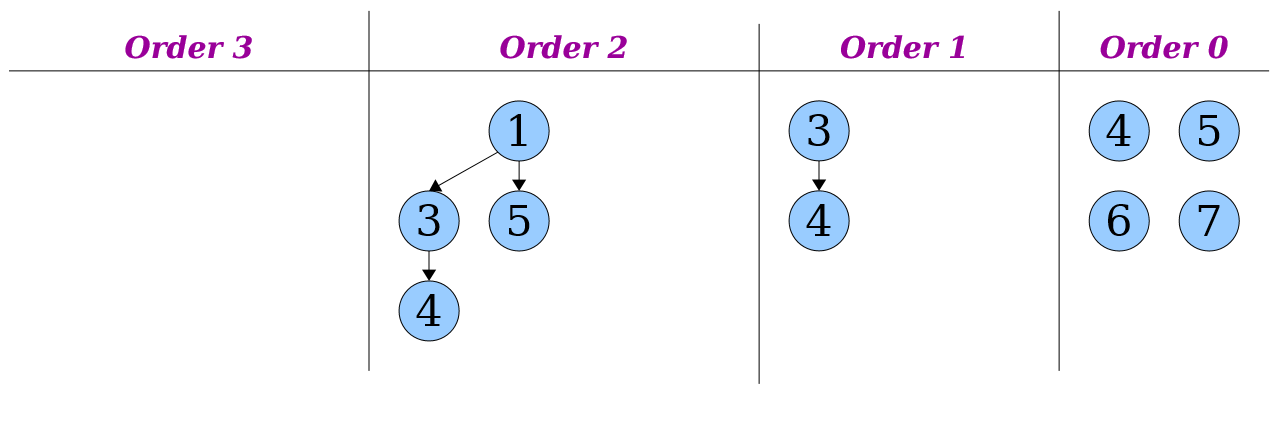先创建 O(logn)个箱子，O(logn)。再 distribute 按 order 所有的 tree，O(t)。再合并所有需要合并的 tree，O(t)。总复杂度 O(t+logn)。
所以此时 extractMin 的实现:
先 remove min 指针所指向 binomial tree 的 root，把 Bk 分裂成 BK-1…B0 再 lazy meld 回来, O(logn)再 coalesce step, O(t+logn)最后 update min 指针, O(logn)
很遗憾，extractMin worst-case 复杂度 O(t+logn) = O(n)。heap 里全是 B0. 而 insert, meld, minimum O(1). 即便我们此时还不考虑 decreaseKey，但依旧是O(logn)，取决于树的高度。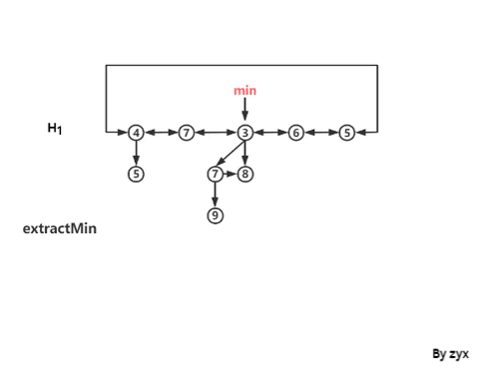The story so far
lazy binomial heap 的 worst-case 性能似乎看起来并不乐观。实际上我们是以牺牲了extractMin 的性能为代价换取了 meld 的高效。那么这个数据结构的均摊复杂度如何呢？
继续使用上次扩充的 potential method.
首先 meld 和 insert worst-case

Θ

(

1

)

\Theta(1)

, amortized 同样

Θ

(

1

)

\Theta(1)

。
Minimum 存了指针，所以依旧

Θ

(

1

)

\Theta(1)

。
最后是关于extractMin的 amortized analysis. 依旧令

Φ

(

S

)

\Phi(S)

表示当前 heap 里 tree 的数量。假设 extractMin 前

Φ

(

S

b

e

f

o

r

e

)

=

t

\Phi(S_{before}) = t

。 actual cost of step 1

=

O

(

log

⁡

n

)

= O(\log n)

actual cost of step 2

=

O

(

t

+

log

⁡

n

)

= O(t+\log n)

actual cost of step 3

=

O

(

log

⁡

n

)

= O(\log n)

（前面都提到过）

Φ

(

S

a

f

t

e

r

)

=

log

⁡

n

\Phi(S_{after}) = \log n

Δ

Φ

=

−

t

+

log

⁡

n

\Delta\Phi = -t + \log n

, actual cost

=

O

(

t

+

log

⁡

n

)

= O(t+\log n)

. 因此“均摊”复杂度 =

O

(

t

+

log

⁡

n

)

+

C

⋅

(

−

t

+

log

⁡

n

)

=

O

(

log

⁡

n

)

O(t+\log n) + C\cdot (-t+\log n) = O(\log n)

最终 lazy binomial heap 的性能如下：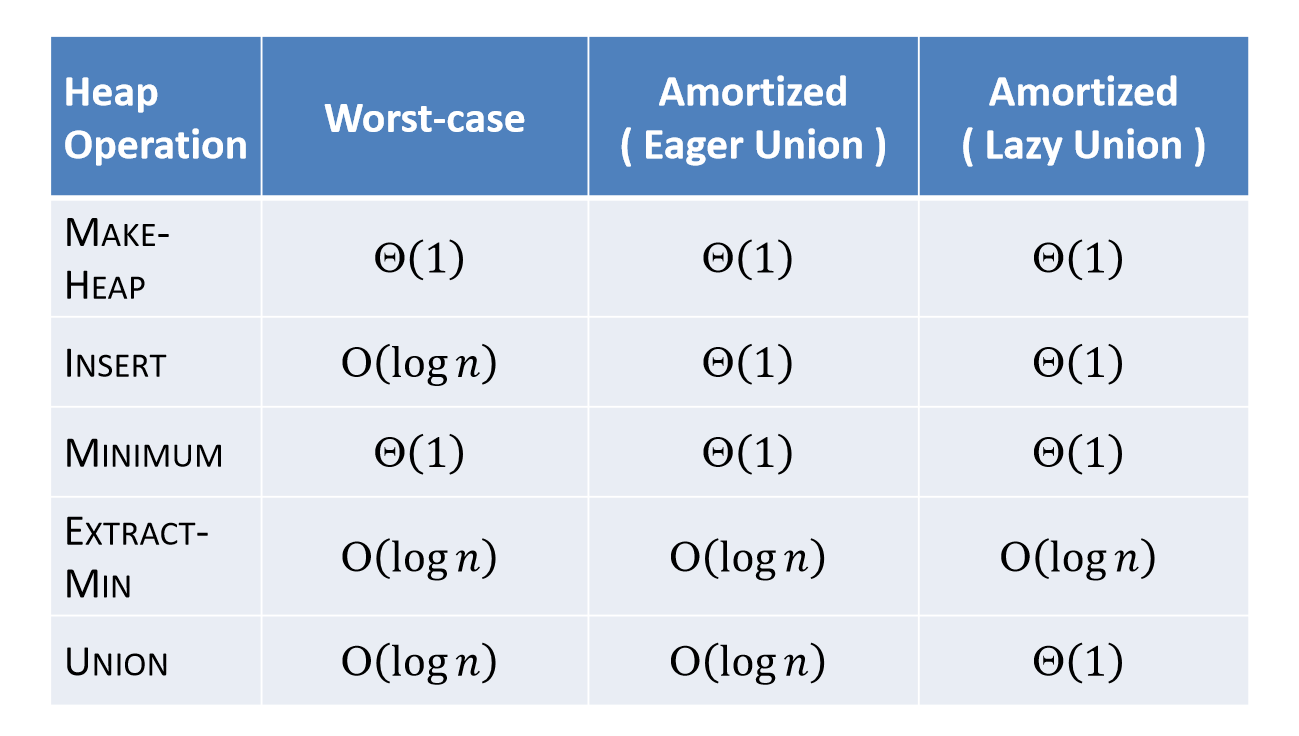（两个性能的图均来自 Stony Brook CSE-548 lecture9）
后记
主要参考了大S的 cs-166，Stony Brook的 CSE-548。
前者会讲很多思路，好像旨在告诉你提出解决办法的人是他们如何思考这个问题的，把你带入到情境中。但是有时候会显得很冗长，啰嗦。而实际上虽然内容很长，200+的slides，但其实并没有披露特别多的细节。而且因为屏蔽了一些细节，所以在一些时候分析的时候就会跟屏蔽细节的结论相悖。
后者很扎实、很干。上来就硬货怼脸的感觉，而且会涉及到比较多的细节，很详尽。可能因为是CSE的缘故…总之如果抱着致知的态度，刨根问底一探究竟的话，极为推荐。可能大S的人都是那种知道顶层思路，享受自己回去自己研究实现的乐趣。确实本来就没有一个正确答案，只要思路对，能实现，细节自己慢慢扣是极好的。
啊…如果本着膜一下名校，去看下CMU的课件，我觉得还是算了吧。看了也白看，内容很少，看了也白看，而且写到 3. Binomial heaps 后面就跟了一句话，To be finished. 在后面就是下一个 lecture的另外的内容了…
最后记一下这些 slides 中的小错。cs-166: performance score find_min

Θ

(

1

)

\Theta(1)

，而在分析时采用的是不维护 min 指针而是去find_min O(t)，感觉没得洗。另外就是太长了…不利于阅读…CSE-548 对于lazy binomial heap 是否维护 min 指针的描述前后矛盾。维护顺带的事儿，不维护的话，O(t)啊！？另外可能会不一致在一些操作的先后顺序是先合并还是先remove没有影响。展开全文队列 数据结构 算法
• Binomial Showdown Time Limit: 1000ms Memory limit: 65536K 有疑问？点这里^_^ 题目描述 In how many ways can you choose k elements out of n elements, not taking order into account? Write...
• var binomial = require ( 'binomial' ) ; binomial ( 4 , 2 ) ; // => 6 binomial ( 10 , 3 ) ; // => 120 执照 麻省理工学院许可证 (MIT) 版权所有 (c) 2014 Olivier Wietrich 特此授予任何人免费获得本软件...JavaScript
• binomial_jonathan:特拉巴里奥决赛C++
• Binomial Showdown（POJ-2249）.pdf
• ## Log binomial 回归详解

千次阅读 2020-12-05 01:45:04
在上一篇，我已经介绍过，可以通过Poisson回归进行分析，除此之外，也可以使用log-binomial回归方法替代logistic回归。Log-binomial 回归模型是广义线性模型的一种特殊类型，由于它很容易得到某一因素率比( rate ...
•R
• var binomial = require ( 'binomial-sampling' ) ; // binomial(n, p) // n is the size of sample // p is the probability of each test console . log ( binomial ( 10 , 0.1 ) ) ; // --> return the ...JavaScript
• 本篇文章轉載於 Binomial Heap - HWCHIU'S BLOG Introduction Binaomial Tree Binomial Heap是由一群 Binomail Tree所組成的Binomial Tree(BT)含有下列特性: 高度為k的 BT共有2^k個node 高度為k的 BT共有2^k個node ...
• 二项堆（Binomial Heap）的c语言完全实现，包括添加，删除，和找到最小值，删除最小值二项堆
• 很清楚的描述了binomial heap 的插入，合并，删除~外国的
• R二项分布检验：双尾二项检验（Two-tailed Binomial Test）、左尾二项检验（Left-tailed Binomial Test）、右尾二项检验.pdf
• B - Binomial coefficients Time Limit:3000MS Memory Limit:0KB 64bit IO Format:%lld & %llu SubmitStatusPracticeUVALive 5900 Description Gunnar is quite an old and forgetful researcher.integer numbers output security 64bit
• eager binomial heaps python实现。使用双向链表，make_heap, insert, merge, find_min, extractMin.
• mainly introduce binary heap,MIN-MAX heaps and binomial heaps. these data structure is very important and interesting.
• 看了很多博客，感觉很多人对np.random.binomial()的解释都写得不是很清楚，或者写错了，或者写得很模糊费解。特别是对该函数的参数解释非常的模糊、不清楚。本文以二项式分布的理解为起点，对该函数进行解释，欢迎...二项式分布
•数据结构 C++
• Lazy binomial heap——python实现前言functionslazy mergeinsertextractMincoalesce_stepupdateMin关于decreaseKey的问题 前言 完整的资源文件和测试代码已经上传。 关于eager binomial heaps的内容详见这篇博客...队列 数据结构 算法导论 算法...